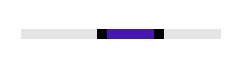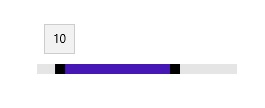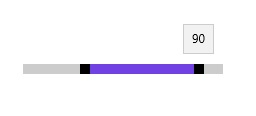# Setting Range in UWP Range Slider (SfRangeSlider)

10 May 20211 minute to read

The SfRangeSlider control supports to select range of value using two Thumbs.

## ShowRange

When ShowRange property is set to true, two Thumbs are placed in the track. One Thumb is used to update the start of the range selection and another thumb is used to update the end of the range selection.

``<editors:SfRangeSlider x:Name="rangeSlider" Width="200" VerticalAlignment="Center" Minimum="0" Maximum="100" ShowRange="True" RangeStart="40" RangeEnd="70"/>``
``rangeSlider.ShowRange = true;``
``rangeSlider.ShowRange = True``## RangeStart

Gets or sets the start value of the range start.

``<editors:SfRangeSlider x:Name="rangeSlider" Width="200" VerticalAlignment="Center" Minimum="0" Maximum="100" ShowRange="True" RangeStart="10" RangeEnd="70"/>``
``rangeSlider.RangeStart = 10;``
``rangeSlider.RangeStart = 10``## RangeEnd

Gets or sets the end value of the range end.

``<editors:SfRangeSlider x:Name="rangeSlider" Width="200" VerticalAlignment="Center" Minimum="0" Maximum="100" ShowRange="True" RangeStart="30" RangeEnd="90"  />``
``rangeSlider.RangeEnd = 90;``
``rangeSlider.RangeEnd = 90``